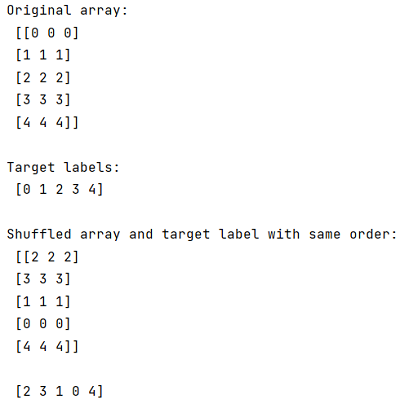# How to randomly shuffle data and target in Python?

In this tutorial, we will learn how to randomly shuffle data and target in Python? By Pranit Sharma Last updated : May 05, 2023

Suppose that we are given with a multi-dimensional array, and with a 2D target label array and we need to randomly shuffle the data by using random.shuffle(), but also, want to keep the labels shuffled by the same order of our array.

## How to randomly shuffle data and target?

To randomly shuffle data and target in Python, first shuffle the indices of the original array within the range of the length of the original array and then we can index the target labels with the shuffled indices and also we can index the original array to shuffle the original array in a consistent way.

Let us understand with the help of an example,

## Python program for randomly shuffle data and target

```# Import numpy
import numpy as np

# Import shuffle
from random import shuffle

# Creating an array
arr = np.array([[0, 0, 0], [1, 1, 1], [2, 2, 2], [3, 3, 3], [4, 4, 4]])

# Display original array
print("Original array:\n",arr,"\n")

# Creating target labels
tar = np.array([0, 1, 2, 3, 4])

# Display target labels
print("Target labels:\n",tar,"\n")

# Shuffling array and target labels by same order
ind = [i for i in range(len(arr))]
shuffle(ind)

arr_new = arr[ind]
tar_new = tar[ind]

# Display result
print("Shuffled array and target label with same order:\n",arr_new,"\n\n",tar_new,"\n")
```

### Output